Six

PROBABILISTIC RETRIEVAL

Introduction

So far in this book we have made very little use of probability theory in modelling any sub-system in IR. The reason for this is simply that the bulk of the work in IR is non-probabilistic, and it is only recently that some significant headway has been made with probabilistic methods[1,2,3]. The history of the use of probabilistic methods goes back as far as the early sixties but for some reason the early ideas never took hold. In this chapter I shall be describing methods of retrieval, i.e. searching and stopping rules, based on probabilistic considerations. In Chapter 2 I dealt with automatic indexing based on a probabilistic model of the distribution of word tokens within a document (text); here I will be concerned with the distribution of index terms over the set of documents making up a collection or file. I shall be relying heavily on the familiar assumption that the distribution of index terms throughout the collection, or within some subset of it, will tell us something about the likely relevance of any given document.

Perhaps it is as well to warn the reader that some of the material in this chapter is rather mathematical. However, I believe that the framework of retrieval discussed in this chapter is both elegant and potentially extremely powerful*. Although the work on it has been rather recent and thus some may feel that it should stand the test of time, I think it probably represents the most important break-through in IR in the last few years. Therefore I unashamedly make this chapter theoretical, since the theory must be thoroughly understood if any further progress is to be made. There are a number of equivalent ways of presenting the basic theory; I have chosen to present it in such a way that connections with other fields such as pattern recognition are easily made. I shall have more to say about other formulations in the Bibliographic Remarks at the end of the chapter.

The fundamental mathematical tool for this chapter is Bayes' Theorem: most of the equations derive directly from it. Although the underlying mathematics may at first look a little complicated the interpretation is rather simple. So, let me try and immediately given some interpretation of what is to follow.

* This was recognised by Maron in his 'The Logic Behind a Probabilistic Interpretation' as early as 1964.

Remember that the basic instrument we have for trying to separate the relevant from the non-relevant documents is a matching function, whether it be that we are in a clustered environment or an unstructured one. The reasons for picking any particular matching function have never been made explicit, in fact mostly they are based on intuitive argument in conjunction with Ockham's Razor. Now in this chapter I shall attempt to use simple probability theory to tell us what a matching function should look like and how it should be used. The arguments are mainly theoretical but in my view fairly conclusive. The only remaining doubt is about the acceptability of the assumptions, which I shall try and bring out as I go along. The data used to fix such a matching function are derived from the knowledge of the distribution of the index terms throughout the collection of some subset of it. If it is defined on some subset of documents then this subset can be defined by a variety of techniques: sampling, clustering, or trial retrieval. The data thus gathered are used to set the values of certain parameters associated with the matching function. Clearly, should the data contain relevance information then the process of defining the matching function can be iterated by some feedback mechanism similar to the one due to Rocchio described in the previous chapter. In this way the parameters of the matching function can be 'learnt'. It is on matching functions derived from relevance information that we shall concentrate.

It will be assumed in the sequel that the documents are described by binary state attributes, that is, absence or presence of index terms. This is not a restriction on the theory, in principle the extension to arbitrary attributes can be worked out, although it is not clear that this would be worth doing.

Estimation or calculation of relevance

When we search a document collection, we attempt to retrieve relevant documents without retrieving non-relevant ones. Since we have no oracle which will tell us without fail which documents are relevant and which are non-relevant we must use imperfect knowledge to guess for any given document whether it is relevant or non-relevant. Without going into the philosophical paradoxes associated with relevance, I shall assume that we can only guess at relevance through summary data about the document and its relationships with other documents. This is not an unreasonable assumption particularly if one believes that the only way relevance can ultimately be decided is for the user to read the full text. Therefore, a sensible way of computing our guess is to try and estimate for any document its probability of relevance

PQ (relevance/document)

where the Q is meant to emphasise that it is for a specific query. It is not clear at all what kind of probability this is (see Good for a delightful summary of different kinds), but if we are to make sense of it with a computer and the primitive data we have, it must surely be one based on frequency counts. Thus our probability of relevance is a statistical notion rather than a semantic one, but I believe that the degree of relevance computed on the basis of statistical analysis will tend to be very similar to one arrived at one semantic grounds. Just as a matching function attaches a numerical score to each document and will vary from document to document so will the probability, for some it will be greater than for others and of course it will depend on the query. The variation between queries will be ignored for now, it only becomes important at the evaluation stage. So we will assume only one query has been submitted to the system and we are concerned with

P (relevance/document).

Let us now assume (following Robertson) that:

(1) The relevance of a document to a request is independent of other documents

in the collection.

With this assumption we can now state a principle, in terms of probability of relevance, which shows that probabilistic information can be used in an optimal manner in retrieval. Robertson attributes this principle to W. S Cooper although Maron in 1964 already claimed its optimality.

The probability ranking principle. If a reference retrieval system's response to each request is a ranking of the documents in the collection in order of decreasing probability of relevance to the user who submitted the request, where the probabilities are estimated as accurately as possible on the basis of whatever data have been made available to the system for this purpose, the overall effectiveness of the system to its user will be the best that is obtainable on the basis of those data.

Of course this principle raises many questions as to the acceptability of the assumptions. For example, the Cluster Hypothesis, that closely associated documents tend to be relevant to the same requests, explicitly assumes the contrary of assumption (1). Goffman too, in his work has gone to some pains to make an explicit assumption of dependence. I quote: 'Thus, if a document x has been assessed as relevant to a query s, the relevance of the other documents in the file X may be affected since the value of the information conveyed by these documents may either increase or decrease as a result of the information conveyed by the document x.' Then there is the question of the way in which overall effectiveness is to be measured. Robertson in his paper shows the probability ranking principle to hold if we measure effectiveness in terms of Recall and Fallout. The principle also follows simply from the theory in this chapter. But this is not the place to argue out these research questions, however, I do think it reasonable to adopt the principle as one upon which to construct a probabilistic retrieval model. One word of warning, the probability ranking principle can only be shown to be true for one query. It does not say that the performance over a range of queries will be optimised, to establish a result of this kind one would have to be specific about how one would average the performance across queries.

The probability ranking principle assumes that we can calculate P(relevance/document), not only that, it assumes that we can do it accurately. Now this is an extremely troublesome assumption and it will occupy us some more further on. The problem is simply that we do not know which are the relevant documents, nor do we know how many there are so we have no way of calculating P(relevance/document). But we can, by trial retrieval, guess at P(relevance/ document) and hopefully improve our guess by iteration. To simplify matters in the subsequent discussion I shall assume that the statistics relating to the relevant and non-relevant documents are available and I shall use them to build up the pertinent equations. However, at all times the reader should be aware of the fact that in any practical situation the relevance information must be guessed at (or estimated).

So returning now to the immediate problem which is to calculate, or estimate, P(relevance/ document). For this we use Bayes' Theorem, which relates the posterior probability of relevance to the prior probability of relevance and the likelihood of relevance after observing a document. Before we plunge into a formal expression of this I must introduce some symbols which will make things a little easier as we go along.

Basic probabilistic model*

Since we are assuming that each document is described by the presence/absence of index terms any document can be represented by a binary vector,

x = (x1,x2, . . ., xn)

where xi = 0 or 1 indicates absence or presence of the ith index term. We also assume that there are two mutually exclusive events,

w1 = document is relevant

w2 = document is non-relevant.

* The theory that follows is at first rather abstract, the reader is asked to bear with it, since we soon return to the

nuts and bolts of retrieval.

So, in terms of these symbols, what we wish to calculate for each document is P(w1/x) and perhaps P(w2/x) so that we may decide which is relevant and which is non-relevant. This is a slight change in objective from simply producing a ranking, we also wish the theory to tell us how to cut off the ranking. Therefore we formulate the problem as a decision problem. Of course we cannot estimate P(wi/x) directly so we must find a way of estimating it in terms of quantities we do know something about. Bayes' Theorem tells us that for discrete distributionsHere P(wi) is the prior probability of relevance (i=1) or non-relevance (i=2), P(x/wi) is proportional to what is commonly known as the likelihood of relevance or non-relevance given x; in the continuous case this would be a density function and we would write p(x/wi). Finally,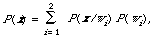which is the probability of observing x on a random basis given that it may be either relevant or non-relevant. Again this would be written as a density function p(x) in the continuous case. Although P(x) (or p(x) ) will mostly appear as a normalising factor (i.e. ensuring that P(w1/x) + P(w2/x) = 1) it is in some ways the function we know most about, it does not require a knowledge of relevance for it to be specified. Before I discuss how we go about estimating the right hand side of Bayes' Theorem I will show how the decision for or against relevance is made.

The decision rule we use is in fact well known as Bayes' Decision Rule. It is

[P (w1/x) > P(w2/x) -> x is relevant, x is non-relevant] * D1

The expression D1 is a short hand notation for the following: compare P (w1/x) with P(w2/x) if the first is greater than the second then decide that x is relevant otherwise decide x is non-relevant. The case P(w1/x) = P(w2/x) is arbitrarily dealt with by deciding non-relevance. The basis for the rule D1 is simply that it minimises the average probability of error, the error of assigning a relevant document as non-relevant or vice versa. To see this note that for any x the probability of error is* The meaning of [E -> p,q] is that if E is true then decide p, otherwise decide q.

In other words once we have decided one way (e.g. relevant) then the probability of having made an error is clearly given by the probability of the opposite way being the case (e.g. non-relevant). So to make this error as small as possible for any given x we must always pick that wi for which P (w1/x) is largest and by implication for which the probability of error is the smallest. To minimise the average probability of error we must minimise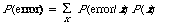This sum will be minimised by making P (error/x) as small as possible for each x since P(error/x) and P(x) are always positive. This is accomplished by the decision rule D1 which now stands as justified.

Of course average error is not the only sensible quantity worth minimising. If we associate with each type of error a cost we can derive a decision rule which will minimise the overall risk. The overall risk is an average of the conditional risks R(wi/x) which itself in turn is defined in terms of a cost function lij. More specifically lij is the loss incurred for deciding wi when wj is the case. Now the associated expected loss when deciding wi is called the conditional risk and is given by

R (wi/x) - li1P(w1/x) + li2P(w2/x) i = 1, 2

The overall risk is a sum in the same way that the average probability of error was, R (wi/x) now playing the role of P(wi/x). The overall risk is minimised by

[R (w1/x) < R (w2/x) -> x is relevant, x is non-relevant] D2

D1 and D2 can be shown to be equivalent under certain conditions. First we rewrite D1, using Bayes' Theorem, in a form in which it will be used subsequently, viz.

[P( x/w1) P (w1) > P( x/w2) P(w2) -> x is relevant, x is non-relevant] D3

Notice that P(x) has disappeared from the equation since it does not affect the outcome of the decision. Now, using the definition R (wi/x) it is easy to show that

[R (w1/x) < R (w2/x) ] [[equivalence]] [(l21 - l11) P( x/w1) P(w1) > (l12 - l22) P( x/w2) P(w2)]

When a special loss function is chosen, namely,which implies that no loss is assigned to a correct decision (quite reasonable) and unit loss to any error (not so reasonable), then we have

[R (w1/x) < R (w2/x) [[equivalence]] P(x/w1) P (w1) > P(x/w2) P(w2)]

which shows the equivalence of D2 and D3, and hence of D1 and D2 under a binary loss function.

This completes the derivation of the decision rule to be used to decide relevance or non-relevance, or to put it differently to retrieve or not to retrieve. So far no constraints have been put on the form of P(x/w1), therefore the decision rule is quite general. I have set up the problem as one of deciding between two classes thereby ignoring the problem of ranking for the moment. One reason for this is that the analysis is simpler, the other is that I want the analysis to say as much as possible about the cut-off value. When ranking, the cut-off value is usually left to the user; within the model so far one can still rank, but the cut-off value will have an interpretation in terms of prior probabilities and cost functions. The optimality of the probability ranking principle follows immediately from the optimality of the decision rule at any cut-off. I shall now go on to be more precise about the exact form of the probability functions in the decision rule.

Form of retrieval function

The previous section was rather abstract and left the connection of the various probabilities with IR rather open. Although it is reasonable for us to want to calculate P(relevance/document) it is not at all clear as to how this should be done or whether the inversion through Bayes' Theorem is the best way of getting at it. Nevertheless, we will proceed assuming that P(x/wi) is the appropriate function to estimate. This function is of course a joint probability function and the interaction between the components of x may be arbitrarily complex. To derive a workable decision rule a simplifying assumption about P(x/wi) will have to be made. The conventional mathematically convenient way of simplifying P(x/wi) is to assume the component variables xi of x to be stochastically independent. Technically this amounts to making the major assumption

P(x/wi) = P(x1/wi) P(x2/wi) ... P(xn/wi) A1

Later I shall show how this stringent assumption may be relaxed. We also for the moment ignore the fact that assuming independence conditional on both w1 and w2 separately has implications about the dependence conditional on w1 [[logicalor]] w2.

Let us now take the simplified form of P(x/wi) and work out what the decision rule will look like. First we define some variables

pi = Prob (xi = 1/w1)

qi = Prob (xi = 1/w2).

In words pi(qi) is the probability that if the document is relevant (non-relevant) that the ith index term will be present. The corresponding probabilities for absence are calculated by subtracting from 1, i.e. 1 - pi = Prob (xi = 0/w1). The likelihood functions which enter into D3 will now look as follows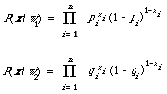To appreciate how these expressions work, the reader should check that P((0,1,1,0,0,1)/w1) = (1 - p1)p2p3(1 - p4)(1 - p5)p6. Substituting for P(x/wi) in D3 and taking logs, the decision rule will be transformed into a linear discriminant function.where the constants ai, bi and e are obvious.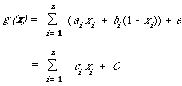andThe importance of writing it this way, apart from its simplicity, is that for each document x to calculate g(x) we simply add the coefficients ci for those index terms that are present, i.e. for those ci for which xi = 1. The ci are often looked up as weights; Robertson and Sparck Jones call ci a relevance weight, and Salton calls exp(ci) the term relevance. I shall simply refer to it as a coefficient or a weight. Hence the name weighting function for g(x).

The constant C which has been assumed the same for all documents x will of course vary from query to query, but it can be interpreted as the cut-off applied to the retrieval function. The only part that can be varied with respect to a given query is the cost function, and it is this variation which will allow us to retrieve more or less documents. To see this let us assume that l11 = l22 = 0 and that we have some choice in setting the ratio l21/l11 by picking a value for the relative importance we attach to missing a relevant document compared with retrieving a non-relevant one. In this way we can generate a ranking, each rank position corresponding to a different ratio l21/l12.

Let us now turn to the other part of g(x), namely ci and let us try and interpret it in terms of the conventional 'contingency' table.There will be one such table for each index term; I have shown it for the index term i although the subscript i has not been used in the cells. If we have complete information about the relevant and non-relevant documents in the collection then we can estimate pi by r/R and qi by (n - r)/(N - R). Therefore g(x) can be rewritten as follows: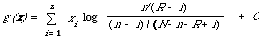This is in fact the weighting formula F4 used by Robertson and Sparck Jones1 in their so called retrospective experiments. For later convenience let us set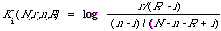There are a number of ways of looking at Ki. The most interesting interpretation of Ki is to say that it measures the extent to which the ith term can discriminate between the relevant and non-relevant documents.

Typically the 'weight' Ki(N,r,n,R) is estimated from a contingency table in which N is not the total number of documents in the system but instead is some subset specifically chosen to enable Ki to be estimated. Later I will use the above interpretation of Ki to motivate another function similar to Ki to measure the discrimination power of an index term.

The index terms are not independent

Although it may be mathematically convenient to assume that the index terms are independent it by no means follows that it is realistic to do so. The objection to independence is not new, in 1964 H. H. Williams expressed it this way: 'The assumption of independence of words in a document is usually made as a matter of mathematical convenience. Without the assumption, many of the subsequent mathematical relations could not be expressed. With it, many of the conclusions should be accepted with extreme caution.' It is only because the mathematics become rather intractable if dependence is assumed that people are quick to assume independence. But, 'dependence is the norm rather than the contrary' to quote the famous probability theorist De Finetti. Therefore the correct procedure is to assume dependence and allow the analysis to simplify to the independent case should the latter be true. When speaking of dependence here we mean stochastic dependence; it is not intended as logical dependence although this may imply stochastic dependence. For IR data, stochastic dependence is simply measured by a correlation function or in some other equivalent way. The assumption of dependence could be crucial when we are trying to estimate P(relevance/document) in terms of P(x/wi) since the accuracy with which this latter probability is estimated will no doubt affect the retrieval performance. So our immediate task is to make use of dependence (correlation) between index terms to improve our estimate of P(x/wi) on which our decision rule rests.

In general the dependence can be arbitrarily complex as the following identity illustrates,

P(x) = P(x1)P(x2/x1)P(x3/x1,x2) ... P(xn/x1,x2, ... , xn - 1)

Therefore, to capture all dependence data we would need to condition each variable in turn on a steadily increasing set of other variables. Although in principle this may be possible, it is likely to be computationally inefficient, and impossible in some instances where there is insufficient data to calculate the high order dependencies. Instead we adopt a method of approximation to estimate P(x) which captures the significant dependence information. Intuitively this may be described as one which looks at each factor in the above expansion and selects from the conditioning variables one particular variable which accounts for most of the dependence relation. In other words we seek a product approximation of the form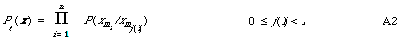where (m1, m2, ..., mn) is a permutation of the integers 1,2, ..., n and j(.) is a function mapping i into integers less than i, and P(xi/xm0) is P(xi). An example for a six component vector x = (x1, ..., x6) might be

Pt(x) = P(x1)P(x2/x1)P(x3/x2)P(x4/x2)P(x5/x2)P (x6/x5)

Notice how similar the A2 assumption is to the independence assumption A1, the only difference being that in A2 each factor has a conditioning variable associated with it. In the example the permutation (m1, m2, ..., m6) is (1,2, ..., 6) which is just the natural order, of course the reason for writing the expansion for Pt(x) the way I did in A2 is to show that a permutation of (1,2, ..., 6) must be sought that gives a good approximation. Once this permutation has been found the variables could be relabelled so as to have the natural order again.

The permutation and the function j(.) together define a dependence tree and the corresponding Pt(x) is called a probability distribution of (first-order) tree dependence. The tree corresponding to our six variable example is shown in Figure 6.1. The tree shows which variable appears either side of the conditioning stroke in P(./.). Although I have chosen to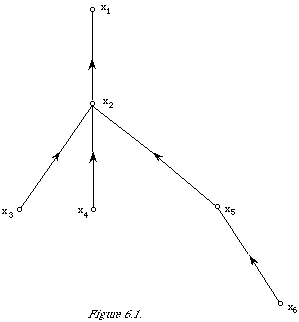write the function Pt(x) the way I did with xi as the unconditioned variable, and hence the root of the tree, and all others consistently conditioned each on its parent node, in fact any one of the nodes of the tree could be singled out as the root as long as the conditioning is done consistently with respect to the new root node. (In Figure 6.1 the 'direction' of conditioning is marked by the direction associated with an edge.) The resulting Pt(x) will be the same as can easily be shown by using the fact thatApplying this to the link between the root node x1 and its immediate descendant x2 in the example will shift the root to x2 and change the expansion to

Pt(x1, x2, ... x6) = P(x2)P(x1)/x2)P(x3/x2)P(x4/x2)P(x5/x2)P (x6/x5)

Of course, to satisfy the rule about relabelling we would exchange the names '1' and '2'. All expansions transformed in this way are equivalent in terms of goodness of approximation to P(x). It is therefore the tree which represents the class of equivalent expansions. Clearly there are a large number of possible dependence trees, the approximation problem we have is to find the best one; which amounts to finding the best permutation and mapping j(.).

In what follows I shall assume that the relabelling has been done and that xmi = xi.

Selecting the best dependence trees

Our problem now is to find a probability function of the form Pt(x) on a set of documents which is the best approximation to the true joint probability function P(x), and of course a better approximation than the one afforded by making assumption A1*. The set on which the approximation is defined can be arbitrary, it might be the entire collection, the relevant documents (w1), or the non-relevant documents (w2). For the moment I shall leave the set unspecified, all three are important. However, when constructing a decision rule similar to D4 we shall have to approximate P(x/w1) and P(x/w2).

The goodness of the approximation is measured by a well known function (see, for example, Kullback); if P(x) and Pa(x) are two discrete probability distributions then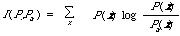* That this is indeed the case is shown by Ku and Kullback.

is a measure of the extent to which Pa(x) approximates P(x). In terms of this function we want to find a distribution of tree dependence Pt(x) such that I(P,Pt) is a minimum. Or to put it differently to find the dependence tree among all dependence trees which will make I(P,Pt) as small as possible.

If the extent to which two index terms i and j deviate from independence is measured by the expected mutual information measure (EMIM) (see Chapter 3, p 41).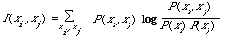then the best approximation Pt(x), in the sense of minimising I(P,Pt), is given by the maximum spanning tree (MST) (see Chapter 3, p.56) on the variables x1, x2, ..., xn . The spanning tree is derived from the graph whose nodes are the index terms 1,2, ..., n, and whose edges are weighted with I(xi,xj). The MST is simply the tree spanning the nodes for which the total weightis a maximum. This is a highly condensed statement of how the dependence tree is arrived at, unfortunately a fuller statement would be rather technical. A detailed proof of the optimisation procedure can be found in Chow and Liu. Here we are mainly interested in the application of the tree structure.

One way of looking at the MST is that it incorporates the most significant of the dependences between the variables subject to the global constraint that the sum of them should be a maximum. For example, in Figure 6.1 the links between the variables (nodes, x1, ..., x6) have been put in just because the sum

I(x1,x2) +I(x2,x3) + I(x2,x4) + I(x2,x5) + I(x5/x6)

is a maximum. Any other sum will be less than or equal to this sum. Note that it does not mean that any individual weight associated with an edge in the tree will be greater than one not in the tree, although this will mostly be the case.

Once the dependence tree has been found the approximating distribution can be written down immediately in the form A2. From this I can derive a discriminant function just as I did in the independent case.

ti = Prob (xi = 1/xj(i) = 1)

ri = Prob (xi = 1/xj(i) = 0) and r1 = Prob (x1 = 1)

P(xi /xj(i)) = [ti[xi](1 - ti) [- xi]] [xj(i) []ri[xi ](1 - ri) [- xi]]  [- xj(i)]

thenThis is a non-linear weighting function which will simplify to the one derived from A1 when the variables are assumed to be independent, that is, when ti = ri. The constant has the same interpretation in terms of prior probabilities and loss function. The complete decision function is of course

g(x) = log P(x/w1) - log P(x/w2)

which now involves the calculation (or estimation) of twice as many parameters as in the linear case. It is only the sum involving xj(i) which make this weighting function different from the linear one, and it is this part which enables a retrieval strategy to take into account the fact that xi depends on xj(i). When using the weighting function a document containing xj(i), or both xi and xj(i), will receive a contribution from that part of the weighting function.

It is easier to see how g(x) combines different weights for different terms if one looks at the weights contributed to g(x) for a given document x for different settings of a pair of variables xi ,xj(i). When xi = 1 and xj(i) = 0 the weight contributed is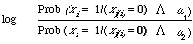and similarly for the other three settings of xi and xj(i).

This shows how simple the non-linear weighting function really is. For example, given a document in which i occurs but j(i) does not, then the weight contributed to g(x) is based on the ratio of two probabilities. The first is the probability of occurrence of i in the set of relevant documents given that j(i) does not occur, the second is the analogous probability computed on the non-relevant documents. On the basis of this ratio we decide how much evidence there is for assigning x to the relevant or non-relevant documents. It is important to remember at this point that the evidence for making the assignment is usually based on an estimate of the pair of probabilities.

Estimation of parameters

The use of a weighting function of the kind derived above in actual retrieval requires the estimation of pertinent parameters. I shall here deal with the estimation of ti and ri for the non-linear case, obviously the linear case will follow by analogy. To show what is involved let me given an example of the estimation process using simple maximum likelihood estimates. The basis for our estimates is the following 2-by-2 table.Here I have adopted a labelling scheme for the cells in which [x] means the number of occurrences in the cell labelled x. Ignoring for the moment the nature of the set on which this table is based; our estimates might be as follows: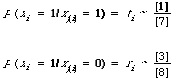In general we would have two tables of this kind when setting up our function g(x), one for estimating the parameters associated with P(x/w1) and one for P(x/w2). In the limit we would have complete knowledge of which documents in the collection were relevant and which were not. Were we to calculate the estimates for this limiting case, this would only be useful in showing what the upper bound to our retrieval would be under this particular model. More realistically, we would have a sample of documents, probably small (not nesessarily random), for which the relevance status of each document was known. This small set would then be the source data for any 2-by-2 tables we might wish to construct. The estimates therefore would be biased in an unavoidable way.

The estimates shown above are examples of point estimates. There are a number of ways of arriving at an appropriate rule for point estimation. Unfortunately the best form of estimation rule is still an open problem. In fact, some statisticians believe that point estimation should not be attempted at all. However in the context of IR it is hard to see how one can avoid making point estimates. One major objection to any point estimation rule is that in deriving it some 'arbitrary' assumptions are made. Fortunately in IR there is some chance of justifying these assumptions by pointing to experimental data gathered from retrieval systems, thereby removing some of the arbitrariness.

Two basic assumptions made in deriving any estimation rule through Bayesian decision theory are:

(1) the form of the prior distribution on the parameter space, i.e. in our case the assumed

probability distribution on the possible values of the binomial parameter; and

(2) the form of the loss function used to measure the error made in estimating the

parameter.

Once these two assumptions are made explicit by defining the form of the distribution and loss function, then, together with Bayes' Principle which seeks to minimise the posterior conditional expected loss given the observations, we can derive a number of different estimation rules. The statistical literature is not much help when deciding which rule is to be preferred. For details the reader should consult van Rijsbergen where further references to the statistical literature are given. The important rules of estimating a proportion p all come in the formwhere x is the number of successes in n trials, and a and b are parameters dictated by the particular combination of prior and loss function. Thus we have a whole class of estimation rules. For example when a=b=0 we have the usual estimate x/n, and when a=b=/2 we have a rule attributed to Sir Harold Jeffreys by Good. This latter rule is in fact the rule used by Robertson and Sparck Jones in their estimates. Each setting of a and b can be justified in terms of the reasonableness of the resulting prior distribution. Since what is found reasonable by one man is not necessarily so for another, the ultimate choice must rest on performance in an experimental test. Fortunately in IR we are in a unique position to do this kind of test.

One important reason for having estimation rules different from the simple x/n, is that this is rather unrealistic for small samples. Consider the case of one sample (n = 1) and the trial result x = 0 (or x = 1) which would result in the estimate for p as p = 0 (or p = 1). This is clearly ridiculous, since in most cases we would already know with high probability that

0 < p < 1. To overcome this difficulty we might try and incorporate this prior knowledge in a distribution on the possible values of the parameter we are trying to estimate. Once we have accepted the feasibility of this and have specified the way in which estimation error is to be measured, Bayes' Principle (or some other principle) will usually lead to a rule different from x/n.

This is really as much as I wish to say about estimation rules, and therefore I shall not push the technical discussion on this points any further; the interested reader should consult the readily accessible statistical literature.

Recapitulation

At this point I should like to summarise the formal argument thus far so that we may reduce it to simple English. One reason for doing this now is that so far I have stuck closely to what one might call a 'respectable' theoretical development. But as in most applied subjects, in IR when it comes to implementing or using a theory one is forced by either inefficiency or inadequate data to diverge from the strict theoretical model. Naturally one tries to diverge as little as possible, but it is of the essence of research that heuristic modifications to a theory are made so as to fit the real data more closely. One obvious consequence is that it may lead to a better new theory.

The first point to make then, is that, we have been trying to estimate P(relevance/document), that is, the probability of relevance for a given document. Although I can easily write the preceding sentence it is not at all clear that it will be meaningful. Relevance in itself is a difficult notion, that the probability of relevance means something can be objected to on the same grounds that one might object to the probability of Newton's Second Law of Motion being the case. Some would argue that the probability is either one or zero depending on whether it is true or false. Similarly one could argue for relevance. The second point is that the probability P(relevance/document) can be got at by considering the inverse probability P(x/relevance), thus relating the two through Bayes' Theorem. It is not that I am questioning the use of Bayes' Theorem when applied to probabilities, which is forced upon us anyhow if we want to use probability theory consistently, no, what I am questioning is that P(x/relevance) means something in IR and hence can lead us to P(relevance/x). I think that we have to assume that it does, and realise that this assumption will enable us to connect P(relevance/x) with the distributional information about index terms.

To approach the problem in this way would be useless unless one believed that for many index terms the distribution over the relevant documents is different from that over the non-relevant documents. If we assumed the contrary, that is P(x/relevance) = P(x/non-relevance) then the P(relevance/document) would be the same as the prior probability of P(relevance), constant for all documents and hence incapable of discriminating them which is of no use in retrieval. So really we are assuming that there is indirect information available through the joint distribution of index terms over the two sets which will enable us to discriminate them. Once we have accepted this view of things then we are also committed to the formalism derived above. The commitment is that we must guess at P(relevance/document) as accurately as we can, or equivalently guess at P(document/relevance) and P(relevance), through the distributional knowledge we have of the attributes (e.g. index terms) of the document.

The elaboration in terms of ranking rather than just discrimination is trivial: the cut-off set by the constant in g(x) is gradually relaxed thereby increasing the number of documents retrieved (or assigned to the relevant category). The result that the ranking is optimal follows from the fact that at each cut-off value we minimise the overall risk. This optimality should be treated with some caution since it assumes that we have got the form of the P(x/wi)'s right and that our estimation rule is the best possible. Neither of these are likely to be realised in practice.

If one is prepared to let the user set the cut-off after retrieval has taken place then the need for a theory about cut-off disappears. The implication is that instead of working with the ratio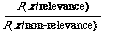we work with the ratio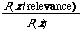In the latter case we do not see the retrieval problem as one of discriminating between relevant and non-relevant documents, instead we merely wish to compute the P(relevance/x) for each document x and present the user with documents in decreasing order of this probability. Whichever way we look at it we still require the estimation of two joint probability functions.

The decision rules derived above are couched in terms of P(x/wi). Therefore one would suppose that the estimation of these probabilities is crucial to the retrieval performance, and of course the fact that they can only be estimated is one explanation for the sub-optimality of the performance. To facilitate the estimation one makes assumptions about the form of P(x/wi). An obvious one is to assume stochastic independence for the components of x. But in general I think this is unrealistic because it is in the nature of information retrieval that index terms will be related to one another. To quote an early paper of Maron's on this point: 'To do this [enlarge upon a request] one would need to program a computing machine to make a statistical analysis of index terms so that the machine will "know" which terms are most closely associated with one another and can indicate the most probable direction in which a given request should be enlarged' [Maron's italics]. Therefore a more realistic approach is to assume some sort of dependence between the terms when estimating P(x/w1) and P(x/w2) (or P(x)).

I will now proceed to discuss ways of using this probabilistic model of retrieval and at the same time discuss some of the practical problems that arise. At first I will hardly modify the model at all. But then I will discuss a way of using it which does not necessarily accord strictly with the assumptions upon which it was built in the first place. Naturally the justification for any of this will lie in the province of experimental tests of which many still remain to be done. But first I shall explain a minor modification arising from the need to reduce the dimensionality of our problem.

The curse of dimensionality

In deriving the decision rules I assumed that a document is represented by an n-dimensional vector where n is the size of the index term vocabulary. Typically n would be very large, and so the dimension of the (binary) document vectors is always likely to be greater than the number of samples used to estimate the parameters in the decision function. That this will lead to problems has been pointed out repeatedly in the pattern recognition literature. Although the analysis of the problem in pattern recognition applies to IR as well, the solutions are not directly applicable. In pattern recognition the problem is: given the number of samples that have been used to 'train' the decision function (our weighting function), is there an optimum number of measurements that can be made of an unknown pattern so that the average probability of correct assignment can be maximised? In our case how many index terms can we legitimately use to decide on relevance. Hughes shows that for a very general probabilistic structure the number of measurements is surprisingly small even though reasonably sized samples are used to 'train' the decision function.

Ideally one would like to be able to choose a (small) subset of index terms to which the weighting function g(.) would be restricted thereby maximising the average probability of correct assignment. In pattern recognition there are complicated techniques for doing just that for the equivalent problem. In information retrieval we are fortunate in that there is a natural way in which the dimensionality of the problem can be reduced. We accept that the query terms are a fair guide to the best features to be used in the application of g(.) to decide between relevance and non-relevance. Therefore rather than computing the weighting function for all possible terms we restrict g(.) to the terms specified in the query and possibly their close associates. This would be as if during the retrieval process all documents are projected from a high dimensional space into a subspace spanned by a small number of terms.

Computational details

I now turn to some of the more practical details of computing g(x) for each x when the variables xi are assumed to be stochastically dependent. The main aim of this section will be to demonstrate that the computations involved are feasible. The clearest way of doing this is to discuss the calculation of each 'object' EMIM, MST, and g(.) separately and in that order.

1. Calculation of EMIM

The calculation of the expected mutual information measure can be simplified. Then EMIM itself can be approximated to reduce the computation time even further. We take the simplification first.

When computing I(xi,xj) for the purpose of constructing an MST we need only to know the rank ordering of the I(xi,xj)'s. The absolute values do not matter. Therefore if we use simple maximum likelihood estimates for the probabilities based on the data contained in the following table (using the same notation as on p.125).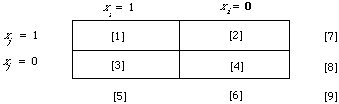then I(xi,xj) will be strictly monotone withThis is an extremely simple formulation of EMIM and easy to compute. Consider the case when it is P(x) we are trying to calculate. The MST is then based on co-occurrence data derived from the entire collection. Once we have this (i.e. ) and know the number of documents () in the file then any inverted file will contain the rest of the frequency data needed to fill in the counts in the other cells. That is from  and  given by the inverted file we can deduce     and .

The problem of what to do with zero entries in one of the cells 1 to 4 is taken care of by letting 0 log 0 = 0. The marginals cannot be zero since we are only concerned with terms that occur at least once in the documents.

Next we discuss the possibility of approximation. Maron and Kuhns in their early work used

d(xi,xj) = P(xi = 1, xj = 1) - P(xi =1) P(xj = 1) (*)

to measure the deviation from independence for any two index terms i and j. Apart from the log this is essentially the first term of the EMIM expansion. An MST (dependence tree) constructed on the basis of (*) clearly would not lead to an optimal approximation of P(x/wi) but the fit might be good enough and certainly the corresponding tree can be calculated more efficiently based on (*) than one based on the full EMIM. Similarly Ivie usedas a measure of association. No doubt there are other ways of approximating the EMIM which are easier to compute, but whether they can be used to find a dependence tree leading to good approximation of the joint probability function must remain a matter for experimental test.

2. Calculation of MST

There are numerous published algorithms for generating an MST from pairwise association measures, the most efficient probably being the recent one due to Whitney. The time dependence of his algorithm is 0(n) where n is the number of index terms to be fitted into the tree. This is not a barrier to its use on large data sets, for it is easy to partition the data by some coarse clustering technique as recommended on p.59, after which the total spanning tree can be generated by applying the MST algorithm to each cluster of index terms in turn. This will reduce the time dependence from 0(n) to 0(k) where k << n.

It is along these lines that Bentley and Friedman have shown that by exploiting the geometry of the space in which the index terms are points the computation time for generating the MST can be shown to be almost always 0(n log n). Moreover if one is prepared to accept a spanning tree which is almost an MST then a computation time of 0(n log n) is guaranteed.

One major inefficiency in generating the MST is of course due to the fact that all n(n-1)/2 associations are computed whereas only a small number are in fact significant in the sense that they are non-zero and could therefore be chosen for a weight of an edge in the spanning tree. However, a high proportion are zero and could safely be omitted. Unfortunately, the only way we can ignore them is to first compute them. Croft in a recent design for the single-link algorithm has discovered a way of ignoring associations without first computing them. It does however presuppose that a file and its inverted form are available, so that if this is not so some computation time would need to be invested in the inversion. It may be that a similar algorithm could be devised for computing an MST.

3. Calculation of g(x)

It must be emphasised that in the non-linear case the estimation of the parameters for g(x) will ideally involve a different MST for each of P(x/w1) and P(x/w2). Of course one only has complete information about the distribution of index terms in the relevant/non-relevant sets in an experimental situation. The calculation of g(x) using complete information may be of interest when deriving upper bounds for retrieval effectiveness under the model as for example was done for the independent case in Robertson and Sparck Jones. In an operational situation where no relevant documents are known in advance, the technique of relevance feedback would have to be used to estimate the parameters repeatedly so that the performance may converge to the upper bound. That in theory the convergence will take place is guaranteed by the convergence theorem for the linear case at least as discussed on p. 106 in Chapter 5. The limitations mentioned there also apply here.

There is a choice of how one would implement the model for g(x) depending on whether one is interested in setting the cut-off a prior or a posteriori. In the former case one is faced with trying to build an MST for the index terms occurring in the relevant documents and one for the ones occurring in the non-relevant documents. Since one must do this from sample information the dependence trees could be far from optimal. One heuristic way of meeting the situation is to construct a dependence tree for the whole collection. The structure of this tree is then assumed to be the structure for the two dependence trees based on the relevant and non-relevant documents. P(x/w1) and P(x/w2) are then calculated by computing the conditional probabilities for the connected nodes dictated by the one dependence tree. How good this particular approximation is can only be demonstrated by experimental test.

If one assumes that the cut-off is set a posteriori then we can rank the documents according to P(w1/x) and leave the user to decide when he has seen enough. In other words we use the form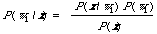to calculate (estimate) the probability of relevance for each document x. Now here we only need to estimate for P(x/w1), since top calculate P(x) we simply use the spanning tree for the entire collection without considering relevance information at all. This second approach has some advantages (ignoring the absence of an explicit mention of cut-off), one being that if dependence is assumed on the entire collection then this is consistent with assuming independence on the relevant documents, which from a computational point of view would simplify things enormously. Although independence on w1 is unlikely it nevertheless may be forced upon us by the fact that we can never get enough information by sampling or trial retrieval to measure the extent of the dependence.

An alternative way of using the dependence tree (Association Hypothesis)

Some of the arguments advanced in the previous section can be construed as implying that the only dependence tree we have enough information to construct is the one on the entire document collection. Let us pursue this line of argument a little further. To construct a dependence tree for index terms without using relevance information is similar to constructing an index term classification. In Chapter 3 I pointed out the relationship between the MST and single-link, which shows that the one is not very different from the other. This leads directly to the idea that perhaps the dependence tree could be used in the same way as one would a term clustering.

The basic idea underlying term clustering was explained in Chapter 2. This could be summarised by saying that based on term clustering various strategies for term deletion and addition can be implemented. Forgetting about 'deletion' for the moment, it is clear how the dependence tree might be used to add in terms to, or expand, the query. The reason for doing this was neatly put by Maron in 1964: 'How can one increase the probability of retrieving a class of documents that includes relevant material not otherwise selected? One obvious method suggests itself: namely, to enlarge the initial request by using additional index terms which have a similar or related meaning to those of the given request'. The assumption here is that 'related meaning' can be discovered through statistical association. Therefore I suggest that given a query, which is an incomplete specification of the information need and hence the relevant documents, we use the document collection (through the dependence tree) to tell us what other terms not already in the query may be useful in retrieving relevant documents. Thus I am claiming that index terms directly related (i.e. connected) to a query term in the dependence tree are likely to be useful in retrieval. In a sense I have reformulated the hypothesis on which term clustering is based (see p.31). Let me state it formally now, and call it the Association Hypothesis:

If an index term is good at discriminating relevant from non-relevant documents then any closely associated index term is also likely to be good at this.

The way we interpret this hypothesis is that a term in the query used by a user is likely to be there because it is a good discriminator and hence we are interested in its close associates. The hypothesis does not specify the way in which association between index terms is to be measured although in this chapter I have made a case for using EMIM. Neither does it specify a measure of 'discrimination', this I consider in the next section. The Association Hypothesis in some ways is a dual to the Cluster Hypothesis (p. 45) and can be tested in the same way.

Discrimination power of an index term

On p. 120 I definedand in fact there made the comment that it was a measure of the power of term i to discriminate between relevant and non-relevant documents. The weights in the weighting function derived from the independence assumption A1 are exactly these Ki's. Now if we forget for the moment that these weights are a consequence of a particular model and instead consider the notion of discrimination power of an index term on its own merits. Certainly this is not a novel thing to do, Salton in some of his work has sought effective ways of measuring the 'discrimination value' of index terms. It seems reasonable to attach to any index term that enters into the retrieval process a weight related to its discrimination power. Ki as a measure of this power is slightly awkward in that it becomes undefined when the argument of the log function becomes zero. We therefore seek a more 'robust' function for measuring discrimination power. The function I am about to recommend for this purpose is indeed more robust, has an interesting interpretation, and enables me to derive a general result of considerable interest in the next section. However, it must be emphasised that it is only an example of a function which enables some sense to be make of the notion 'discrimination power' in this and the next section. It should therefore not be considered unique although it is my opinion that any alternative way of measuring discrimination power in this context would come very close to the measure I suggest here.

Instead of Ki I suggest using the information radius, defined in Chapter 3 on p. 42, as a measure of the discrimination power of an index term. It is a close cousin of the expected mutual information measure a relationship that will come in useful later on. Using u and v as positive weights such as u + v = 1 and the usual notation for the probability functions we can write the information radius as follows:The interesting interpretation of the information radius that I referred to above is illustrated most easily in terms of continuous probability functions. Instead of using the densities p(./w1) and p(./w2) I shall use the corresponding probability measure u1 and u2. First we define the average of two directed divergencies,

R (u1, u2/v) = uI (u1/v) +vI (u2/v)

where I(ui/v) measures the expectation on ui of the information in favour of rejecting v for ui given by making an observation; it may be regarded as the information gained from being told to reject v in favour of ui. Now the information radius is the minimum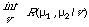thereby removing the arbitrary v. In fact it turns out that the minimum is achieved when

v = u u1 + v u2

that is, an average of the two distributions to be discriminated. If we now adopt u and v as the prior probabilities then v is in fact given by the density

p(x) = p(x/w1) P(w1) + p(x/w2) P(w2)

defined over the entire collection without regard to relevance. Now of this distribution we are reasonably sure, the distribution u1 and u2 we are only guessing at; therefore it is reasonable when measuring the difference between u1 and u2 that v should incorporate as much of the information that is available. The information radius does just this.

There is one technical problem associated with the use of the information radius, or any other 'discrimination measure' based on all four cells of the contingency table, which is rather difficult to resolve. As a measure of discrimination power it does not distinguish between the different contributions made to the measure by the different cells. So, for example, an index term might be a good discriminator because it occurs frequently in the non-relevant documents and infrequently in the relevant documents. Therefore, to weight an index term proportional to the discrimination measure whenever it is present in a document is exactly the wrong thing to do. It follows that the data contained in the contingency table must be used when deciding on a weighting scheme.

Discrimination gain hypothesis

In the derivation above I have made the assumption of independence or dependence in a straightforward way. I have assumed either independence on both w1 and w2, or dependence. But, as implied earlier, this is not the only way of making these assumptions. Robertson and Sparck Jones make the point that assuming independence on the relevant and non-relevant documents can imply dependence on the total set of documents. To see this consider two index terms i and j, and

P(xi, xj) = P(xi, xj /w1)P(w1) + P(xi, xi /w2) P (w2)

P(xi) P( xj) = [P(xi /w1)P(w1) + P(xi, w2) P (w2)] [P(xj /w1) P(w1) + P(xj,w2) P (w2)]

If we assume conditional independence on both w1 and w2 then

P(xi, xj) = P(xi, /w1) P(xj, w1) P(w1) + P(xi /w2) P(xj/ w2) P (w2)

For unconditional independence as well, we must have

P(xi, xj) = P(xi) P(xj)

This will only happen when P(w1) = 0 or P(w2) = 0, or P(xi/ w1) = P(xi/w2), or P(xj/w1) = P(xj /w2), or in words, when at least one of the index terms is useless at discriminating relevant from non-relevant documents. In general therefore conditional independence will imply unconditional dependence. Now let us assume that the index terms are indeed conditionally independence then we get the following remarkable results.

Kendall and Stuart define a partial correlation coefficient for any two distributions bywhere [[rho]] (.,./W) and [[rho]] (.,.) are the conditional and ordinary correlation coefficients respectively. Now if X and Y are conditionally independent then

[[rho]] (X, Y/W) = 0

which implies using the expression for the partial correlation that

[[rho]] (X, Y) = [[rho]] (X, W) [[rho]] (Y, W)

Since

| [[rho]] (X, Y) | <= 1 , | [[rho]] (X, W) | <= 1 , | [[rho]] (Y, W) | <= 1

this in turn implies that under the hypothesis of conditional independence

| [[rho]] (X, Y) | < | [[rho]] (X, W) | or | [[rho]] (Y, W) | (**)

Hence if W is a random variable representing relevance then the correlation between it and either index term is greater than the correlation between the index terms.

Qualitatively I shall try and generalise this to functions other than correlation coefficients, Linfott defines a type of informational correlation measure by

rij = (1 - exp (-2I (xi, xj) ) )[1/2 ]0 <= rij <= 1

orwhere I (xi, xj) is the now familiar expected mutual information measure. But rij reduces to the standard correlation coefficient [[rho]] (.,.) if (xi, xj) is normally distributed. So it is not unreasonable to assume that for non-normal distributions rij will behave approximately like [[rho]] (.,.) and will in fact satisfy (**) as well. But rij is strictly monotone with respect to I (x,i, xj) so it too will satisfy (**). Therefore we can now say that under conditional independence the information contained in one index term about another is less than the information contained in either term about the conditioning variable W. In symbols we have

I (xi, xj) < I (xi, W) or I (xj, W),

where I (., W) is the information radius with its weights interpreted as prior probabilities. Remember that I (.,W) was suggested as the measure of discrimination power. I think this result deserves to be stated formally as an hypothesis when W is interpreted as relevance.

Discrimination Gain Hypothesis: Under the hypothesis of conditional independence the statistical information contained in one index term about another is less than the information contained in either index term about relevance.

I must emphasise that the above argument leading to the hypothesis is not a proof. The argument is only a qualitative one although I believe it could be tightened up. Despite this it provides (together with the hypothesis) some justification and theoretical basis for the use of the MST based on I (xi, xj) to improve retrieval. The discrimination hypothesis is a way of firming up the Association Hypothesis under conditional independence.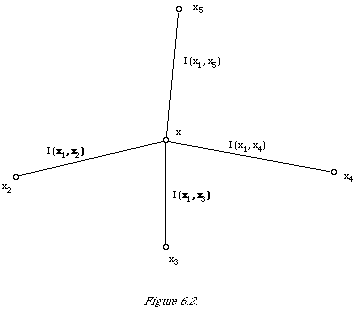One consequence of the discrimination hypothesis is that it provides a rationale for ranking the index terms connected to a query term in the dependence tree in order of I(term, query term) values to reflect the order of discrimination power values. The basis for this is that the more strongly connected an index term is to the query term (measured by EMIM) the more discriminatory it is likely to be. To see what is involved more clearly I have shown an example set-up in Figure 6.2. Let us suppose that x1 is the variable corresponding to the query term and that I (x1, x2) < I (x1, x3) < I (x1, x4) < I (x1, x5) then our hypothesis says that without knowing in advance how good a discriminator each of the index terms 2,3,4,5 is, it is reasonable to assume that I (x2, W) < I (x3, W) < I (x4, W) <I (x5, W). Clearly we cannot guarantee that the index terms will satisfy the last ordering but it is the best we can do given our ignorance.

Bibliographic remarks

The basis background reading for this chapter is contained in but a few papers. One approach to probabilistic weighting based on relevance data derives from the work of Yu and his collaborators[28,29]. The other is contained in the already frequently cited paper of Robertson and Sparck Jones. Unfortunately, both these approaches rely heavily on the assumption of stochastic independence. My own paper and the one of Bookstein and Kraft are the only ones I know of, which try and construct a model without this assumption. Perhaps an earlier paper by Negoita should be mentioned here which discusses an attempt to use non-linear decision functions in IR. Robertson's recent progress in documentation on models gives a useful summary of some of the more recent work.

According to Doyle (p.267), Maron and Kuhns were the first to describe in the open literature the use of association (statistical co-occurrence) of index terms as a means of enlarging and sharpening the search. However, Doyle himself was already working on similar ideas in the late fifties and produced a number of papers on 'associations' in the early sixties[34,35]. Stiles in 1961, already apparently aware of Maron and Kuhns work, gave an explicit procedure for using terms co-occurring significantly with search terms, and not unlike the method based on the dependence tree described in this chapter. He also used the [[chi]] to measure association between index terms which is mathematically very similar to using the expected mutual information measure, although the latter is to be preferred when measuring dependence (see Goodman and Kruskal for a discussion on this point). Stiles was very clear about the usefulness of using associations between index terms, he saw that through them one was 'able to locate documents relevant to a request even though the document had not been indexed by the term used in the request'.

The model in this chapter also connects with two other ideas in earlier research. One is the idea of inverse document frequency weighting already discussed in Chapter 2. The other is the idea of term clustering. Taking the weighting idea first, this in fact goes back to the early paper by Edmundson and Wyllys, we can write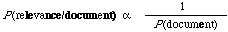or in words, for any document the probability of relevance is inversely proportional the probability with which it will occur on a random basis. If the P(document) is assumed to be the product of the probabilities of the individual index terms being either present or absent in the document then after taking logs we have the inverse document frequency weighting principle. It assumes that the likelihood P(document/relevance) is constant for all documents. Why it is exactly that this principle works so well is not yet clear (but see Yu and Salton's recent theoretical paper).

The connection with term clustering was already made earlier on in the chapter. The spanning tree can be looked upon as a classification of the index terms. One of the important consequences of the model described in this chapter is that it lays down precisely how the tree should be used in retrieval. Earlier work in this area was rather ad hoc and did not lead to conclusive results.

It should be clear now that the quantitative model embodies within one theory such diverse topics as term clustering, early association analysis, document frequency weighting, and relevance weighting.

References

1. ROBERTSON, S.E. and SPARCK JONES, K., 'Relevance weighting of search terms', Journal of the American Society for Information Science, 27, 129-146 (1976)

2. van RIJSBERGEN, C.J., 'A theoretical basis for the use of co-occurrence data in information retrieval', Journal of Documentation, 33, 106-119 (1977).

3. BOOKSTEIN, A. and KRAFT, D., 'Operations research applied to document indexing and retrieval decisions', Journal of the ACM, 24, 410-427 (1977).

4. MARON, M.E., 'Mechanized documentation: The logic behind a probabilistic interpretation', In: Statistical Association Methods for Mechanized Documentation (Edited by Stevens et al.) National Bureau of Standards, Washington, 9-13 (1965).

5. OSBORNE, M.L., 'A Modification of Veto Logic for a Committee of Threshold Logic Units and the Use of 2-class Classifiers for Function Estimation', Ph.D. Thesis, Oregon State University (1975).

6. GOOD, I.J., Probability and the Weighting of Evidence, Charles Griffin and Co.Ltd., London (1950).

7. ROBERTSON, S.E., 'The probability ranking principle in IR', Journal of Documentation, 33, 294-304 (1977).

8. GOFFMAN, W., 'A searching procedure for information retrieval', Information Storage and Retrieval, 2, 294-304 (1977).

9. WILLIAMS, J.H., 'Results of classifying documents with multiple discriminant functions', In : Statistical Association Methods for Mechanized Documentation (Edited by Stevens et al.) National Bureau of Standards, Washington, 217-224 (1965).

10. DE FINETTI, B., Theory of Probability, Vol. 1, 146-161, Wiley, London (1974).

11. KU, H.H. and KULLBACK, S., 'Approximating discrete probability distributions', IEEE Transactions on Information Theory, IT-15, 444-447 (1969).

12. KULLBACK, S., Information Theory and Statistics, Dover, New York (1968).

13. CHOW, C.K. and LIU, C.N., 'Approximating discrete probability distributions with dependence trees', IEEE Transactions on Information Theory, IT-14, 462-467 (1968).

14. COX, D.R., 'The analysis of multivariate binary data', Applied Statistics, 21, 113-120 (1972).

15. BOX, G.E.P. and TIAO, G.C., Bayesian Inference in Statistical Analysis, 304-315, Addison-Wesley, Reading, Mass. (1973).

16. GOOD, I.J., The Estimation of Probabilities: An Essay on Modern Bayesian Methods, The M.I.T. Press, Cambridge, Mass. (1965).

17. HARPER, D. and van RIJSBERGEN, C.J., 'An evaluation of feedback in document retrieval using co-occurrence data', Journal of Documentation, 34, 189-216 (1978).

18. HUGHES, G.F., 'On the mean accuracy of statistical pattern recognizers', IEEE Transactions on Information Theory, IT-14, 55-63 (1968).

19. MARON, M.E. and KUHNS, J.L., 'On relevance, probabilistic indexing and information retrieval', Journal of the ACM, 7, 216-244 (1960).

20. IVIE, E.L., 'Search Procedures Based on Measures of Relatedness Between Documents', Ph.D. Thesis, M.I.T., Report MAC-TR-29 (1966).

21. WHITNEY, V.K.M., 'Minimal spanning tree, Algorithm 422', Communications of the ACM, 15, 273-274 (1972).

22. BENTLEY, J.L. and FRIEDMAN, J.H., Fast Algorithm for Constructing Minimal Spanning Trees in Coordinate Spaces, Stanford Report, STAN-CS-75-529 (1975).

23. CROFT, W.B., 'Clustering large files of documents using single link', Journal of the American Society for Information Science, 28, 341-344 (1977).

24. SALTON, G., Dynamic Information and Library Processing, Prentice-Hall, Englewoods Cliffs, NJ., 441-445 (1975).

25. JARDINE, N. and SIBSON, R., Mathematical Taxonomy, pp. 12-15, Wiley, London and New York (1971).

26. KENDALL, M.G. and STUART, A., Advanced Theory of Statistics, Vol. 2, 2nd ed., Griffin, London (1967).

27. LINFOOT, E.H., 'An informational measure of correlation', Information and Control, 1, 85-89 (1957).

28. YU, C.T. and SALTON, G., "Precision Weighting - An effective automatic indexing method', Journal of the ACM, 23, 76-85 (1976).

29. YU, C.T., LUK, W.S. and CHEUNG, T.Y., 'A statistical model for relevance feedback in information retrieval', Journal of the ACM, 23, 273-286 (1976).

30. NEGOITA, C.V., 'On the decision process in information retrieval', Studii si cercetari de documentare, 15, 269-281 (1973).

31. ROBERTSON, S.E., 'Theories and models in information retrieval', Journal of Documentation, 33, 126-148 (1977).

32. DOYLE, L.B., Information Retrieval and Processing, Melville Publishing Co., Los Angeles, California (1975).

33. DOYLE, L.B., 'Programmed interpretation of text as a basis for information retrieval systems', In: Proceedings of the Western Joint Computer Conference, San Francisco, 60-63 (1959).

34. DOYLE, L.B., 'Semantic road maps for literature searchers', Journal of the ACM, 8, 553-578 (1961).

35. DOYLE, L.B., 'Some compromises between word grouping and document grouping', In: Statistical Association Methods for Mechanized Documentation (Edited by Stevens et al.) National Bureau of Standards, Washington, 15-24 (1965).

36. STILES, H.F., 'The association factor in information retrieval', Journal of the ACM, 8, 271-279 (1961).

37. GOODMAN, L. and KRUSKAL, W., 'Measures of association for cross-classifications', Journal of the American Statistical Association, 49, 732-764 (1954).

38. EDMUNDSON, H.P. and WYLLYS, R.E., 'Automatic abstracting and indexing - Survey and recommendations', Communications of the ACM, 4, 226-234 (1961).

39. YU, C.T. and SALTON, G., 'Effective information retrieval using term accuracy', Communications of the ACM, 20, 135-142 (1977).

40. SPARCK JONES, K., Automatic Keyword Classification for Information Retrieval, Butterworths, London (1971).